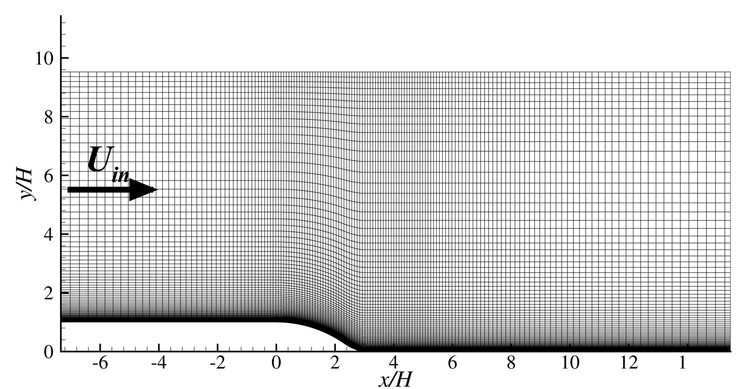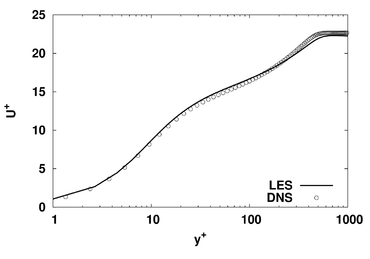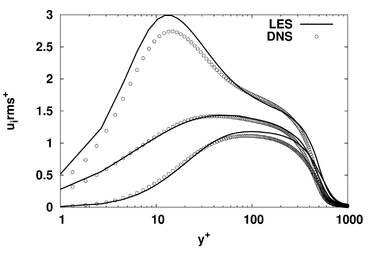Test Case Study

Brief Description of the Study Test Case

The geometry under consideration is shown in Fig. 2. The rounded ramp of height ${H}$is placed in a high-aspect-ratio duct with upstream height of ${8.52H}$. In the simulations, the flow is assumed to be spanwise homogeneous, with the spanwise slab being ${3.7H}$. The assumption of homogeneity is justified by the fact that the experimental ratio of duct depth to the step height was 38. In the experiment [‌31], tripped boundary layers were allowed to develop on both walls for a distance of about ${25H}$. The Reynolds number, based on $H$and the inlet free-stream velocity ${U_{in}}$, is 13,700. At ${x/H=-7.4}$, the computational inlet, the momentum-thickness Reynolds number is ${Re_{\theta }=1192}$, and the boundary-layer thickness is ${\delta _{99}=0.83H}$.

Figure 2: Flow domain and grid (every 8th point shown, fine mesh).The step geometry is based on that used originally by Song and Eaton [‌27]. In order to enlarge the separated region, the height of the step was increased by a factor of 1.5. This adaptation was undertaken interactively with a parallel experiment by Zhang and Zhong [‌31]. The step shape is described by the following three relations, with the origin ${x/H=0}$being the upstream edge of the ramp:

{\begin{aligned}y_{wall}&=(1-R_{1})+{\sqrt {R_{1}^{2}-x_{2}}}\qquad \mathrm {for} \qquad 0with ${R_{1}=4.03}$, ${\ R_{2}=0.333}$, ${\ x_{2}=3.449}$and ${\ y_{2}=1.936}$and ${\ y_{wall}=1}$for ${\ x/H<0}$, ${\ y_{wall}=0}$for ${\ x/H>2.937}$.

Test Case Experiments

The experimental working section is 5.49m long and 1.2m wide. The ramp height is 31.5mm, with an upstream section 660m long. The incoming flow velocity is fixed at 6.5m/s. Thirty-six pressure tapings are made along the ramp between ${x=-400mm}$and 90mm with a spacing of 10mm or 20mm. To prevent an interference with the LDA measurement on the central streamwise plane of the ramp, the pressure tapings are located 25mm off this plane. Combined with the existing pressure tapings on the ceiling of the working section downstream of the ramp (150mm in spacing), the coverage of the pressure measurement is extended further up to 600mm. The velocity at the inlet of the test section is monitored using a Pitot tube. The pressure measurement system consists of a low-range pressure transducer and a scani-valve with forty-eight ports. The pressure sensor has a pressure range of -2.5mbar to 2.5mbar and an accuracy of 0.25% full scale span. The system is remotely controlled by a computer equipped with a data acquisition card. The pressure data are sampled at 1kHz and 10,000 data points are used to produce the time-averaged pressure at each tapping.

A three-component Dantec LDA system is used to measure the boundary layer development around the ramp-down section in both the streamwise and the spanwise direction. The laser beam generated by the 5W Argon ion laser is separated by a series of beam separators and colour filters inside the optic transmitter box to produce three pairs of beams with a wavelength of 512nm, 488nm and 476nm respectively. The averaged sampling rate in the freestream is about 1kHz and a lower rate of about 100Hz is obtained in the near-wall region. The number of realisations used to obtain the time-averaged velocity and turbulence fluctuations is set at 10K, and the time threshold for acquiring the data at each point is 30 seconds. The Dantec 3D traverse specially designed for this LDA system has a resolution of 6.25μm in the three directions. The uncertainty of measurements is about 4 ×10-4 m/s in the freestream and 1.6 ×10-3 m/s in the near-wall region. All velocity components and all Reynolds stresses have been obtained, and the skin-friction is deduced from the LDA velocity measurements, assuming the first point being in the viscous sub-layer. The experimental study is described in detail in [‌31].

CFD Methods

Large-Eddy Simulations

Numerical treatment

The implicitly filtered LES momentum and continuity equations for incompressible flow were solved over a general non-orthogonal, boundary-fitted, multi-block finite volume mesh, using LES-STREAM (e.g. [‌7] for more details and related references). In total, the default mesh covering the duct-flow domain contained around 24 million nodes. The sensitivity to the resolution is addressed below. The variables are stored in a co-located manner. The solution is based on a fractional-step time-marching method, with the time derivative approximated by a third-order Gear scheme [‌7]. The fluxes are approximated by second-order centred approximations. Within the fractional-step algorithm, a provisional velocity field results from advancing the solution with the flux operators. This is then corrected through the pressure field by projecting the provisional solution onto a divergence-free velocity field. To this end, the pressure is computed as a solution to the pressure-Poisson problem with the aid of a V-cycle multigrid scheme. In order to suppress unphysical oscillations, associated with pressure-velocity decoupling, a practice equivalent to that introduced in [‌23] is adopted. Fishpool & Leschziner [‌7] demonstrate that the loss of accuracy associated with the smoothing introduced by this practice is minimal. The subgrid-scale stresses were approximated using either the dynamic Smagorinsky model of [‌10] and [‌16] or the mixed-time scale model of [‌11], the latter being local, involving no averaging and hence being computationally cheaper. The mixed-time scale model was adopted in the additional two grid-sensitivity simulations for the baseline case, and it is the one used for the results presented in the database. The rationale of using the simpler mixed-time scale model for the grid-sensitivity study is rooted primarily in the observation that this model gave somewhat lower time-averaged SGS viscosity values than the dynamic model, and thus allowed any grid-sensitivity specifically associated with variations in resolution to emerge with greater clarity.

Computation Grid $(nx,ny,nz)$$x/H$(separation) $x/H$(reattachment)
Fine (768,160,192) 0.83 4.36
Coarse (448,128,192) 0.87 4.21
Table 1: Grid resolution and separation and reattachment positions

To explore the sensitivity to grid resolution, two simulations were performed (Table 1). The separated region (from ${x/H=0}$to ${x/H=4}$) was resolved using 130 points in the streamwise direction for the coarse mesh, and 280 points in the fine mesh. In both grids, the first grid-cell centre is located below ${y^{+}=1}$at the bottom wall, whether $y$is scaled with the local or inflow friction velocity. The maximum streamwise cell distance, ${x^{+}}$, occurs close to the computational inlet and is around 35. This value drops to below 20 and 10 for the coarse and fine mesh, respectively, downstream of ${x/H=0}$, with values of order 5 within the separation zone. The maximum spanwise cell dimension, ${z^{+}}$, is 10 for both the coarse and fine simulations (cf. [‌3] for more details).

Downstream of separation, the ratio of maximum cell dimension to the Kolmogorov length (derived from the dissipation rate as part of the budget) is of order 6 for the fine grid and 11 for the coarse grid. The boundary layer on the upper wall was covered by a coarser grid than that on the lower wall, with the conditions between the wall and the wall-nearest ${y^{+}}$location of around 25 being covered by a log-law wall function, and with about 17 grid nodes covering the boundary-layer thickness. This choice is justified by the fact that the upper boundary layer is far away from the region of interest, is only subjected to a weak adverse pressure gradient and is always fully attached.

Turbulent boundary layers were imposed at the domain inlet (${x/H=-7.4}$) on both the upper and lower walls. To this end, data were taken from a precursor simulation, performed with the aid of a recycling method [‌17], for a stretch of a boundary layer developing in the actual channel. At a streamwise position at which ${Re_{\theta }=1190}$, every realization of the velocity field was stored over a period of ${1120H/U_{in}}$. This data set was then used to explicitly prescribe the inlet condition. Comparisons between lower-wall boundary-layer data returned by the present precursor simulation and DNS results of Schlatter et al [‌25], for a similar Reynolds number, ${Re_{\theta }=1400}$, are shown on Fig. 3. The present plots pertain to a location immediately downstream of the domain inlet, while Fig. 3 in [‌3] shows distribution derived from the precursor simulation. The slight difference relative to flat-plate boundary-layer data is due to a non-zero streamwise pressure extending from the curved step upstream towards the inlet.

 Figure 3: Mean flow and normal Reynolds stress profiles at the inflow. DNS data from [‌25], for ${Re_{\theta }=1400}$.The time step corresponds to a maximum cell-CFL value of around 0.5. Simulations were run for 300 time units, one time unit being ${H/U_{in}}$, before statistical sampling commenced. The flow was then computed for further 1030 time units during which data for various statistical properties were gathered. Statistical convergence was increased by spanwise averaging.

Data provided

In the database provided for this test case, all quantities have been non-dimensionalized using the step height $H$and the inflow free-stream velocity ${U_{in}}$. The database contains three types of files, all extracted from the fine grid Large-Eddy simulation:

1. wallQuantities.dat contains boundary-layer data computed on the bottom wall. The file contains the following columns:
x, y_wall, C_p, tau_w, delta∗, theta, delta_99
where x${\equiv x/H}$, y_wall is given by Eq. 1),

and

{\begin{aligned}\mathrm {C\_p} &={\frac {p-p_{0}}{{\frac {1}{2}}\rho U_{in}^{2}}}\\\mathrm {tau\_w} &={\frac {1}{Re_{in}}}\left.{\frac {\partial U}{\partial n}}\right|_{y=0}\\\mathrm {delta*} &=\int _{y_{\min }}^{\infty }\left(1-{\frac {U-U_{\min }}{U_{in}-U_{\min }}}\right)dy\\\mathrm {theta} &=\int _{y_{\min }}^{\infty }{\frac {U-U_{\min }}{U_{in}-U_{\min }}}\left(1-{\frac {U-U_{\min }}{U_{in}-U_{\min }}}\right)dy\end{aligned}}where $p_{0}$is taken at the bottom left corner of the domain and delta_99 is the distance to the wall for which ${U=0.99U_{in}}$. ${U_{\min }}$is computed at each streamwise location by ${U_{\min }=\min(U(y),0)}$, for ${y_{wall}\leq y\leq y_{\infty }}$.

1. profiles.dat contains the velocity and Reynolds-Stress profiles in the vertical direction:
x, y, U, V, W, u', v', w', u'v', u'w', v'w'
for 28 different streamwise locations (${x/H}$= -7.31 (first cell center from the inflow), -7, -6, -5, -4, -3, -2, -1, 0, 0.5, 1, 1.5, 2, 2.5, 3, 3.5, 4, 4.5, 5, 6, 7, 8, 9, 10, 11, 12, 13 and 14).

2. balance.#.dat (with # = k, uu, vv, ww or uv) contains the budgets for $\ k$, ${\overline {uu}}$, ${\overline {vv}}$, ${\overline {ww}}$and ${\overline {uv}}$respectively at the same 28 streamwise locations as in profiles.dat. The different terms in these files are given by:

 col Abbrev. $k$-equation ${\overline {u_{i}u_{j}}}$equations 3 convec. ${U_{i}{\frac {\partial k}{\partial x_{i}}}}$${U_{k}{\frac {\partial {\overline {u_{i}u_{j}}}}{\partial x_{k}}}}$4 prod. ${-{\overline {u_{i}u_{j}}}{\frac {\partial U_{i}}{\partial x_{j}}}}$${-{\overline {u_{i}u_{k}}}{\frac {\partial U_{j}}{\partial x_{k}}}-{\overline {u_{j}u_{k}}}{\frac {\partial U_{i}}{\partial x_{k}}}}$5 t-diff. ${-{\frac {1}{2}}{\frac {\partial {\overline {u_{i}u_{i}u_{k}}}}{\partial x_{k}}}}$${-{\frac {\partial {\overline {u_{i}u_{j}u_{k}}}}{\partial x_{k}}}}$6 p-diff. ${-{\frac {1}{2}}{\frac {\partial {\overline {u_{i}p}}}{\partial x_{i}}}}$${-\left({\frac {\partial {\overline {u_{i}p}}}{\partial x_{j}}}+{\frac {\partial {\overline {u_{j}p}}}{\partial x_{i}}}\right)}$7 p-str. ${\ 0}$${{\overline {p{\frac {\partial u_{i}}{\partial x_{j}}}}}+{\overline {p{\frac {\partial u_{j}}{\partial x_{i}}}}}}$8 v-diff. ${{\frac {1}{Re_{in}}}{\frac {\partial ^{2}k}{\partial x_{k}\partial x_{k}}}}$${{\frac {1}{Re_{in}}}{\frac {\partial ^{2}{\overline {u_{i}u_{j}}}}{\partial x_{k}\partial x_{k}}}}$9 diss. ${\ -(3)+(4)+(5)+(6)+(8)}$${\ -(3)+(4)+(5)+(6)+(7)+(8)}$Reynolds-Averaged Navier-Stokes computations

The objective of the RANS computations presented in this document is to guide CFD practitioners and modellers for computing the present step curved flow. All RANS computations have been performed by the author at CD-adapco with a commercial CFD software (STAR-CCM+, ver. 7.02), using the default models, and the results should be easily reproduced using other CFD software. Note that better results have been obtained by using specific modification of the models (e.g. low-Re damping modification of the $\omega$equation, or curvature correction), but the objective here is not to show the best possible results, rather to provide a set of comparison for the most classical formulations.

The four different turbulence models are the standard low-Re ${k-\varepsilon }$model of Launder and Sharma [‌14] but with an alternative damping function [‌15], the realizable ${k-\varepsilon }$model [‌26] used in a two-layer approach (originally introduced in [‌24] as an alternative to low-Reynolds number approach) in which the epsilon equation is not solved near the wall but the turbulent length-scale is specified as a function of the wall-distance, the standard ${k-\omega }$model [‌30], and the SST ${k-\omega }$model [‌19]. Detailed formulation of each model can be found in the STAR-CCM+ documentation and is not repeated here. Note for instance that a low-Re damping of $\omega$(available as an option), while very much applicable to this flow configuration, has not been selected here as it might not be available as standard in all CFD packages. The purpose of this part is purely to draw attention to some particulars when computing this type of spatially developping flow using standard statistical methods.

The grid is the same structured grid as that used in the fine LES described in the previous section, only in 2D because of the spanwise homogeneity. The resolution is thus quite fine, and several tests with coarser grids have shown that the results are grid-independent. The near-wall flow is fully resolved , with ${y^{+}\approx 1}$at the inlet. Note that due to the very thin separated region, high-Reynolds number models are not applicable for this test case: computations with high-Reynolds number models return very small or no separation at all.

Spatially developping flows are very sensitive to the definition of the boundary conditions and most notably to the inflow condition. For all computations, the outflow boundary is treated as a pressure outlet, with a negligible impact on the results if the position of the outflow is located at ${x/H>14}$. For the top wall, two options have been tested: a free-slip or a no-slip condition. The only condition which needs specific attention is the inflow. Different specifications of the inflow length-scale have been tested, only the first (and simplest) one has been used in this report, but details on setting-up the second one are given here. The velocity and turbulence-quantity profiles at the inlet are given by:

1. the velocity vector (only ${U}$and ${V}$are necessary) are extracted from the LES database, at ${x/H=-7.31}$,
2. the turbulent kinetic energy profile at ${x/H=-7.31}$is also extracted from the same database,
3. The length-scale quantity is more problematic and cannot be directly extracted from the database (except for a priori testing. The turbulent eddy-viscosity is over-estimated, which in turn leads to a too high production of the turbulent kinetic energy immediately downstream of the inlet. It was thus decided to impose a constant value for both $\varepsilon$and $\omega$at the inlet, and to allow the flow to develop downstream. The choice of the inlet value for these parameters will thus be dictated by only one requirement: matching the streamwise evolution of the wall-shear stress (Cf. Fig. 7) from ${x/H=-4}$, ie. around ${5H}$upstream of the separation point.

The second method is similar to that used for the large-eddy simulation: it requires a pre-computation of a spatially developing flow on a flat-plate, to determine all the correct quantities at the inflow. A third method would be to extract an eddy-viscosity profile directly from the database and use it at the inflow.

Contributed by: Sylvain Lardeau — CD-adapco, London, UK